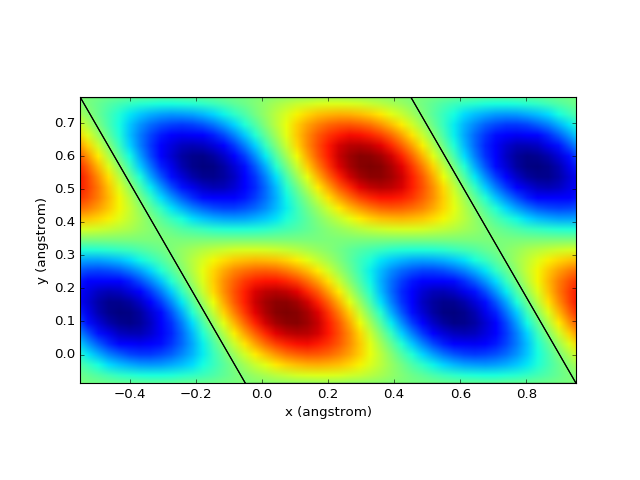# Examples¶

To run the examples you have to install all recommended packages, see corresponding section.

## Atomic structure¶

With DFT Tools you can manipulate crystal structures easily: only very few lines of code required.

### Example: Si unit cell¶

```from dfttools.types import Basis, UnitCell
from dfttools.presentation import svgwrite_unit_cell

from numericalunits import angstrom as a

si_basis = Basis((3.9*a/2, 3.9*a/2, 3.9*a/2, .5,.5,.5), kind = 'triclinic')
si_cell = UnitCell(si_basis, (.5,.5,.5), 'Si')
svgwrite_unit_cell(si_cell, 'output.svg', size = (440,360), show_cell = True)
```One can obtain a supercell by repeating the unit cell:

```mult_cell = si_cell.repeated(3,3,3)
svgwrite_unit_cell(mult_cell, 'output2.svg', size = (440,360), show_cell = True)
```Arbitrary supercell is available via the corresponding function:

```cubic_cell = si_cell.supercell(
(1,-1,1),
(1,1,-1),
(-1,1,1),
)
svgwrite_unit_cell(cubic_cell, 'output3.svg', size = (440,360), show_cell = True, camera = (1,1,1))
```A slab is prepared easily:

```slab_cell = cubic_cell.repeated(5,5,3).isolated(0,0,10*a)
svgwrite_unit_cell(slab_cell, 'output4.svg', size = (440,360), camera = (1,1,1))
```### Example: Monolayer MoS2 line defect¶

A more complex example: monolayer MoS2 with a line defect:

```from dfttools.types import Basis, UnitCell
from dfttools.presentation import svgwrite_unit_cell

from numericalunits import angstrom as a

mos2_basis = Basis(
(3.19*a, 3.19*a, 20*a, 0,0,.5),
kind = 'triclinic'
)
d = 1.57722483162840/20

# Unit cell with 3 atoms
mos2_cell = UnitCell(mos2_basis, (
(1./3,1./3,.5),
(2./3,2./3,0.5+d),
(2./3,2./3,0.5-d),
), ('Mo','S','S'))

# Rectangular supercell with 6 atoms
mos2_rectangular = mos2_cell.supercell(
(1,0,0),
(-1,2,0),
(0,0,1)
)

# Rectangular sheet with a defect
mos2_defect = mos2_rectangular.normalized()
mos2_defect.discard((mos2_defect.values == "S") * (mos2_defect.coordinates[:,1] < .5) * (mos2_defect.coordinates[:,2] < .5))

# Prepare a sheet
mos2_sheet = UnitCell.stack(*((mos2_rectangular,)*3 + (mos2_defect,) + (mos2_rectangular,)*3), vector = 'y')

# Draw
svgwrite_unit_cell(mos2_sheet.repeated(10,1,1), 'output.svg', size = (440,360), camera = (1,1,0.3), camera_top = (0,0,1))
```### Example: parsing structure data¶

It is also possible to obtain atomic structure from the supported files. In this particular case the file source and format can be determined automatically (OpenMX input file).

```from dfttools.presentation import svgwrite_unit_cell
from dfttools.simple import parse

# Parse
with open("plot.py.data", "r") as f:
cell = parse(f, "unit-cell")

# Draw
svgwrite_unit_cell(cell, 'output.svg', size = (440,360), camera = (1,0,0))
```### Example: Moire pattern¶

The Moire pattern is obtained using `UnitCell.supercell`.

```from dfttools.types import Basis, UnitCell
from dfttools.presentation import svgwrite_unit_cell

from numericalunits import angstrom as a

graphene_basis = Basis(
(2.46*a, 2.46*a, 6.7*a, 0,0,.5),
kind = 'triclinic'
)

# Unit cell
graphene_cell = UnitCell(graphene_basis, (
(1./3,1./3,.5),
(2./3,2./3,.5),
), ('C','C'))

# Moire matching vectors
moire = [1, 26, 6, 23]

# A top layer
l1 = graphene_cell.supercell(
(moire,moire,0),
(-moire,moire+moire,0),
(0,0,1)
)

# A bottom layer
l2 = graphene_cell.supercell(
(moire,moire,0),
(-moire,moire+moire,0),
(0,0,1)
)

# Make the basis fit
l2.vectors[:2] = l1.vectors[:2]

# Draw
svgwrite_unit_cell(l1.stack(l2, vector='z'), 'output.svg', size = (440,360), camera = (0,0,-1), camera_top = (0,1,0), show_atoms = False)
```## Band structure¶

The band structures can be easily plotted directly from the output files.

### Example: OpenMX¶

In this case to retrieve the band structure we import parser `dfttools.parsers.openmx.bands` explicitly.

```from dfttools.parsers.openmx import bands
from dfttools import presentation

from matplotlib import pyplot

with open("plot.py.data",'r') as f:

# Plot bands
presentation.matplotlib_bands(b,pyplot.gca())
pyplot.show()
```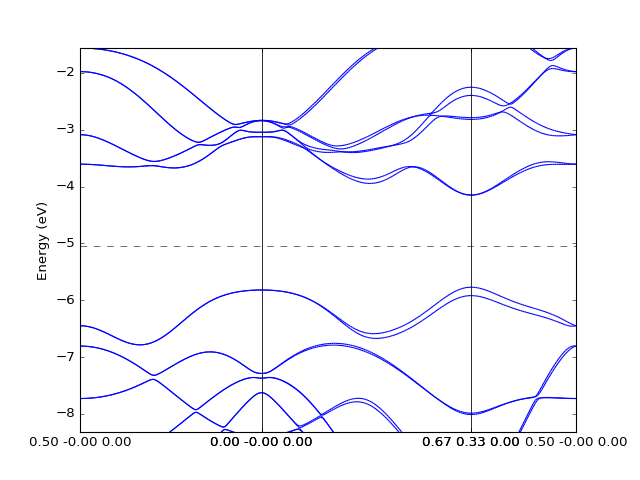### Example: Quantum Espresso¶

The Quantum Espresso files can be identified automatically via `dfttools.simple.parse` routine.

```from dfttools.simple import parse
from dfttools import presentation

from matplotlib import pyplot

with open("plot.py.data",'r') as f:

bands = parse(f, "band-structure")

# Plot bands
presentation.matplotlib_bands(bands,pyplot.gca())
pyplot.show()
```The density of states can be plotted directly from the band structure. However, one has to note that the density calculated from a k-point path is usually not the relevant one.

```from dfttools.simple import parse
from dfttools import presentation

from matplotlib import pyplot

with open("plot.py.data",'r') as f:

bands = parse(f, "band-structure")

# Prepare axes
ax_left  = pyplot.subplot2grid((1,3), (0, 0), colspan=2)
ax_right = pyplot.subplot2grid((1,3), (0, 2))

# Plot bands
presentation.matplotlib_bands(bands,ax_left)
presentation.matplotlib_bands_density(bands, ax_right, 100, orientation = 'portrait')
ax_right.set_ylabel('')
pyplot.show()
```### Example: Density of states¶

To plot an accurate density of states (DoS) a large enough grid is required. Following is an example of a density of states of graphene.

```from dfttools.types import Basis, Grid
from dfttools import presentation

from matplotlib import pyplot
from numericalunits import eV
import numpy

# A reciprocal basis
basis = Basis((1,1,1,0,0,-0.5), kind = 'triclinic', meta = {"Fermi": 0})

# Grid shape
shape = (50,50,1)

# A dummy grid with correct grid coordinates and empty grid values
grid = Grid(
basis,
tuple(numpy.linspace(0,1,x, endpoint = False)+.5/x for x in shape),
numpy.zeros(shape+(2,), dtype = numpy.float64),
)

# Calculate graphene band
k = grid.cartesian()*numpy.pi/3.**.5*2
e = (1+4*numpy.cos(k[...,1])**2 + 4*numpy.cos(k[...,1])*numpy.cos(k[...,0]*3.**.5))**.5*eV

# Set the band values
grid.values[...,0] = -e
grid.values[...,1] = e

presentation.matplotlib_bands_density(grid, pyplot.gca(), 200, energy_range = (-1, 1))
pyplot.show()
```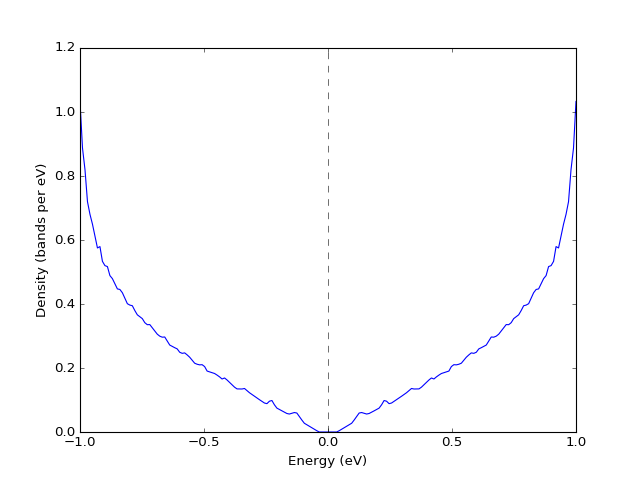### Example: K-point grids: density of states and interpolation¶

They key point of presenting the density of states from a file is converting the band structure to grid via `UnitCell.as_grid`. This only works if you indeed calculated band energies on a grid. Note that while both `Grid` and `UnitCell` can be used for DoS, the former one is considerably more accurate.

```from dfttools.simple import parse
from dfttools import presentation

from matplotlib import pyplot

with open("plot.py.data",'r') as f:

# Retrieve the last band structure from the file
bands = parse(f, "band-structure")

# Convert to a grid
grid = bands.as_grid()

# Plot both
presentation.matplotlib_bands_density(bands, pyplot.gca(), 200, energy_range = (-2, 2), label = "bands")
presentation.matplotlib_bands_density(grid,  pyplot.gca(), 200, energy_range = (-2, 2), label = "grid")
pyplot.legend()
pyplot.show()
```One can also plot the bands by interpolating data on the grid. The quality of the figure depends on the grid size and interpolation methods.

```from dfttools.simple import parse
from dfttools import presentation

import numpy
from matplotlib import pyplot

with open("plot.py.data",'r') as f:

# Retrieve the last band structure from the file
bands = parse(f, "band-structure")

# Convert to a grid
grid = bands.as_grid()

# Interpolate
kp_path = numpy.linspace(0,1)[:,numpy.newaxis] * ((1./3,2./3,0),)
bands = grid.interpolate_to_cell(kp_path)

# Plot
presentation.matplotlib_bands(bands, pyplot.gca())
pyplot.show()
```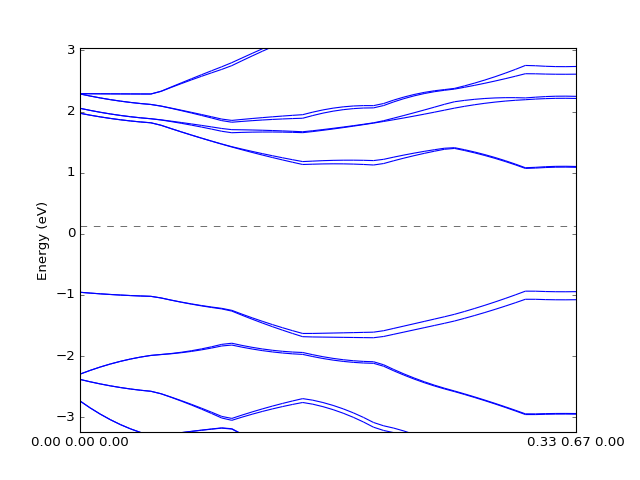### Example: Band structure with weights¶

The band structure with weights is plotted using `weights` keyword argument. The weights array is just numbers assigned to each k-point and each band.

```from dfttools.types import Basis, UnitCell
from dfttools import presentation

from matplotlib import pyplot
from numericalunits import eV
import numpy

# A reciprocal basis
basis = Basis((1,1,1,0,0,-0.5), kind = 'triclinic', meta = {"Fermi": 0})

# G-K path
kp = numpy.linspace(0,1,100)[:,numpy.newaxis] * numpy.array(((1./3,2./3,0),))

# A dummy grid UnitCell with correct kp-path
bands = UnitCell(
basis,
kp,
numpy.zeros((100,2), dtype = numpy.float64),
)

# Calculate graphene band
k = bands.cartesian()*numpy.pi/3.**.5*2
e = (1+4*numpy.cos(k[...,1])**2 + 4*numpy.cos(k[...,1])*numpy.cos(k[...,0]*3.**.5))**.5*eV

# Set the band values
bands.values[...,0] = -e
bands.values[...,1] = e

# Assign some weights
weights = bands.values.copy()
weights -= weights.min()
weights /= weights.max()

# Prepare axes
ax_left  = pyplot.subplot2grid((1,3), (0, 0), colspan=2)
ax_right = pyplot.subplot2grid((1,3), (0, 2))

# Plot bands
p = presentation.matplotlib_bands(bands,ax_left,weights = weights)
presentation.matplotlib_bands_density(bands, ax_right, 100, orientation = 'portrait')
presentation.matplotlib_bands_density(bands, ax_right, 100, orientation = 'portrait', weights = weights, use_fill = True, color = "#AAAAFF")

ax_right.set_ylabel('')
pyplot.colorbar(p)
pyplot.show()
```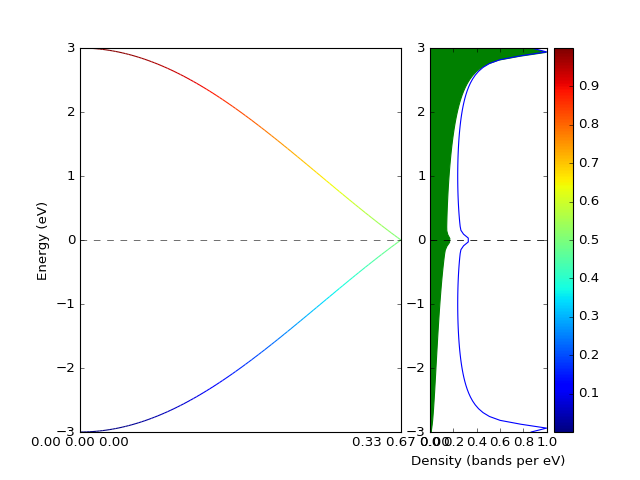## Data on the grid¶

Plotting of data (charge, potential, density, etc.) on a 3D grid is very straightforward.

```from dfttools.types import Basis, Grid
from dfttools import presentation

from numericalunits import angstrom
from matplotlib import pyplot
import numpy

grid = Grid(
Basis((1*angstrom,1*angstrom,1*angstrom,0,0,-0.5), kind = 'triclinic'),
(
numpy.linspace(0,1,30,endpoint = False),
numpy.linspace(0,1,30,endpoint = False),
numpy.linspace(0,1,30,endpoint = False),
),
numpy.zeros((30,30,30)),
)
grid.values = numpy.prod(numpy.sin(grid.explicit_coordinates()*2*numpy.pi), axis = -1)

presentation.matplotlib_scalar(grid, pyplot.gca(), (0.1,0.1,0.1), 'z', show_cell = True)
pyplot.show()
```